Courses

# Coordination Number and Atomic Packing Factor Civil Engineering (CE) Notes | EduRev

## Civil Engineering (CE) : Coordination Number and Atomic Packing Factor Civil Engineering (CE) Notes | EduRev

The document Coordination Number and Atomic Packing Factor Civil Engineering (CE) Notes | EduRev is a part of the Civil Engineering (CE) Course Engineering Physics - Notes, Videos, MCQs & PPTs.
All you need of Civil Engineering (CE) at this link: Civil Engineering (CE)

Coordination number and Atomic packing factor Definition

(1) Coordination number:
It is the number of equidistant nearest neighbors that an atom has in a crystal structure.

(2) Nearest neighbour distance:
It is the distance between two nearest neighbors in a crystal structure.

(3) Atomic packing factor (APF) or Packing fraction:
It is the fraction of space occupied by atoms in a unit cell. It is defined as the ratio of volume occupied by atoms in unit cell to the volume of the unit cell. If the number of atoms per unit cell are n and if Va is the volume of atoms in the unit cell and V is the volume of the unit cell then,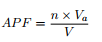Calculation of Atomic packing factor for different crystal systems
Simple Cubic Structure

In simple cubic structure each atom consists of 6 equidistant nearest neighbours. Hence its co-ordination number is 6.
Eight unit cells share each atom at the corner. Hence only 1/8th of the volume of the atom lies in each cell. Since the atoms are present only at corners, the number of atoms per unit cell is given by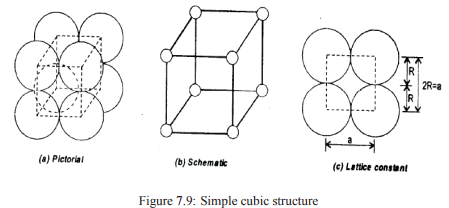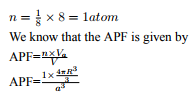In this structure the atoms touch each other along the sides of the cube. Therefore a=2R,where R is the radius of each atom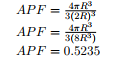Hence atoms occupy 52.35% off the volume of the unit cell.
Body Centered Cubic (BCC) Structure:
Each atom has 8 equidistant nearest neighbors. Hence the co-ordination number is 8. Since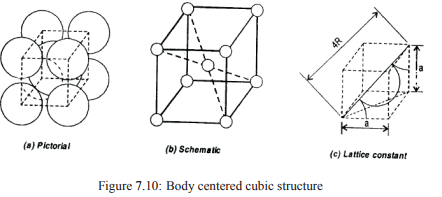there are eight atoms at corners and 1 atom at the body center, the no of atoms per unit cell is given by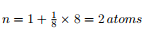Also in this structure the atoms touch each other along the body diagonal. Therefore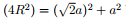Where R is the radius of the atom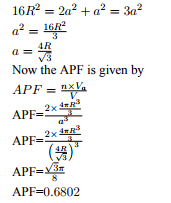Hence atoms occupy 68.02% of the volume of the unit
Face Centered Cubic (FCC) Structure:
In FCC structure in addition to atoms at corners, atoms are present at face centers. Each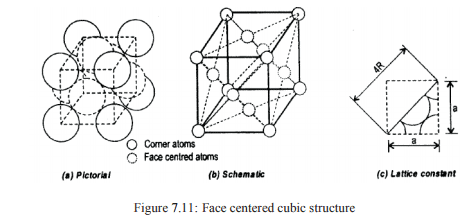atom consists of 12 equidistant nearest neighbours. Hence the coordination number is 12. The number of atoms per unit cell is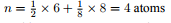In this structure atoms touch each other along the face diagonal. Therefore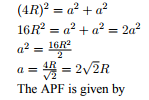Where R is the atomic radius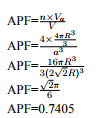Hence atoms occupy 74.05% of the volume of the unit cell.

Offer running on EduRev: Apply code STAYHOME200 to get INR 200 off on our premium plan EduRev Infinity!

,

,

,

,

,

,

,

,

,

,

,

,

,

,

,

,

,

,

,

,

,

;